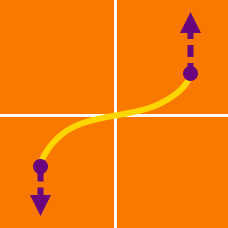Calculus

# Arc Length by Integration

If ${f}'(x) = \sqrt{ {x}^4-1 },$ what is the length of the curve $y = f(x)$ from $x = 2$ to $x = 8?$

What is the length of the curve $y = \frac{1}{2}( {e}^x+{e}^{-x} )$ from $x = -13$ to $x = 13$?

Let $L$ be the length of the line $y = 3x + 4$ on the interval $3 \leq x \leq 8$. If $L = \sqrt{a}$, then what is the value of $a$?

Given $y \geq 0 ,$ what is the length of the curve ${y}^2 = {x}^3$ on the interval $0 \leq x \leq 4$?

Let $L$ be the length of the curve $\displaystyle{y = \frac{1}{4}{x}^2 - \frac{1}{2} \ln x }$ for $4 \leq x \leq 8.$ If $L = a+\frac{1}{2}\ln{b} ,$ where $a$ and $b$ are positive integers, what is the value of $a+b?$

×